# 17 Units

 17 Units
As soon as you’re dealing with real-world quantities, it’s inevitable that you’ll run into units. In the Wolfram Language, you can enter quantities with units using ctrl+=.
Enter a quantity of time in units of hours:
Quantity[2.6, "Hours"]
Quantity[2.6, "Hours"]
Press the check mark to accept the interpretation:
Quantity[2.6, "Hours"]
You can use InputForm to see how the Wolfram Language internally represents this.
Show the internal form of a quantity:
InputForm[Quantity[2.6, "Hours"]]

You can always enter quantities directly like this, or you can use ctrl+=, either for the whole thing, or just for the units.
The Wolfram Language knows about all the 10,000 or so common kinds of units. UnitConvert converts between them.
Convert from hours to minutes:
UnitConvert[Quantity[2.6, "Hours"], "Minutes"]

You can do arithmetic with quantities even if they have different units.
Add a length in feet to one in centimeters:
Quantity[7.5, "Feet"] + Quantity[14, "Centimeters"]

Divide one length by another:
Quantity[7.5, "Feet"]/Quantity[14, "Centimeters"]

Use dollars in a computation:
7.5*Quantity[3., "USDollars"] + 2.51*Quantity[8., "USDollars"]

Multiply a price per pound by a weight in kilograms:
Quantity[15, "USDollars"/"Pounds"]*Quantity[5.6, "Kilograms"]

You can convert between currencies. The Wolfram Language always knows the latest conversion rate.
CurrencyConvert[Quantity[100., "Euros"], Quantity[1, "USDollars"]]

Display a string rotated by 30 degrees:
Rotate["hello", 30 °]

If you leave off the Degree or °, the Wolfram Language will assume you’re talking about radians, which go from 0 to 2π (about 6.28) around a circle, rather than degrees, which go from 0 to 360.
π/2 radians is equivalent to 90°:
Rotate["hello", Pi/2]

Make a list of rotations in degrees from 0 to 360:
Table[Rotate[n, n Degree], {n, 0, 360, 30}]

There’s lots to do with angles. For example, AnglePath gives the path you’d follow if you successively turned by a sequence of angles.
Start off horizontal, then turn three times by 80°:
Graphics[Line[AnglePath[{0 °, 80 °, 80 °, 80 °}]]]

Keep turning by 80° and you’ll eventually get back to where you started:
Graphics[Line[AnglePath[Table[80 °, 20]]]]

If you keep increasing the angle, you get an interesting pattern:
Graphics[Line[AnglePath[Table[n*5 °, {n, 200}]]]]

17.1Convert 4.5 lbs (pounds) to kilograms. »
Expected output:
 Out[]=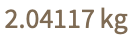Answer & check your solution
17.2Convert 60.25 mph to kilometers per hour. »
Expected output:
 Out[]=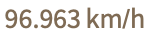Answer & check your solution
17.3Find the height of the Eiffel Tower in miles. »
Expected output:
 Out[]=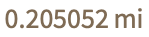Answer & check your solution
17.4Find the height of Mount Everest divided by the height of the Eiffel Tower. »
Expected output:
 Out[]=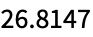Answer & check your solution
17.5Find the mass of the Earth divided by the mass of the Moon. »
Expected output:
 Out[]=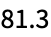Answer & check your solution
17.6Convert 2500 Japanese yen to US dollars. »
Sample expected output:
 Out[]=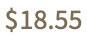Answer & check your solution
17.7Find the total of 35 ounces, 1/4 ton, 45 lbs and 9 stone in kilograms. »
Expected output:
 Out[]=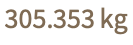Answer & check your solution
17.8Get a list of the distances to each planet using the "DistanceFromEarth" property, and convert all the results to light minutes. »
Expected output:
 Out[]=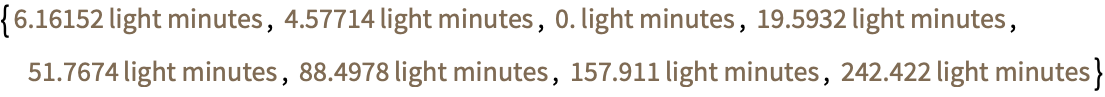Answer & check your solution
17.9Rotate the string "hello" by 180°»
Expected output:
 Out[]=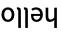Answer & check your solution
17.10Make a table of a size-100 “A” rotated by 0° through 360° in steps of 30°»
Expected output:
 Out[]=Answer & check your solution
17.11Make a Manipulate to rotate an image of a cat between 0° and 180°»
Sample expected output:
 Out[]=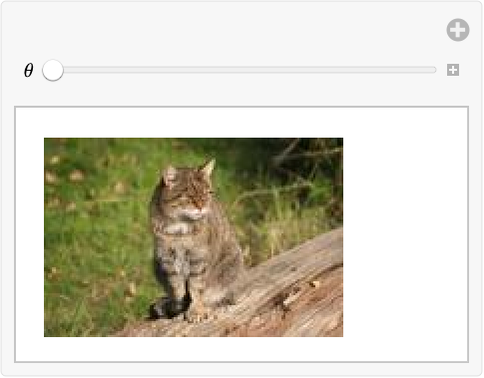Answer & check your solution
17.12Generate graphics for a path obtained by turning 0°, 1°, 2°, ... , 180°»
Expected output:
 Out[]=Answer & check your solution
17.13Make graphics of the path obtained by turning a constant angle 100 times, controlling the angle from 0° to 360° with a Manipulate»
Sample expected output:
 Out[]=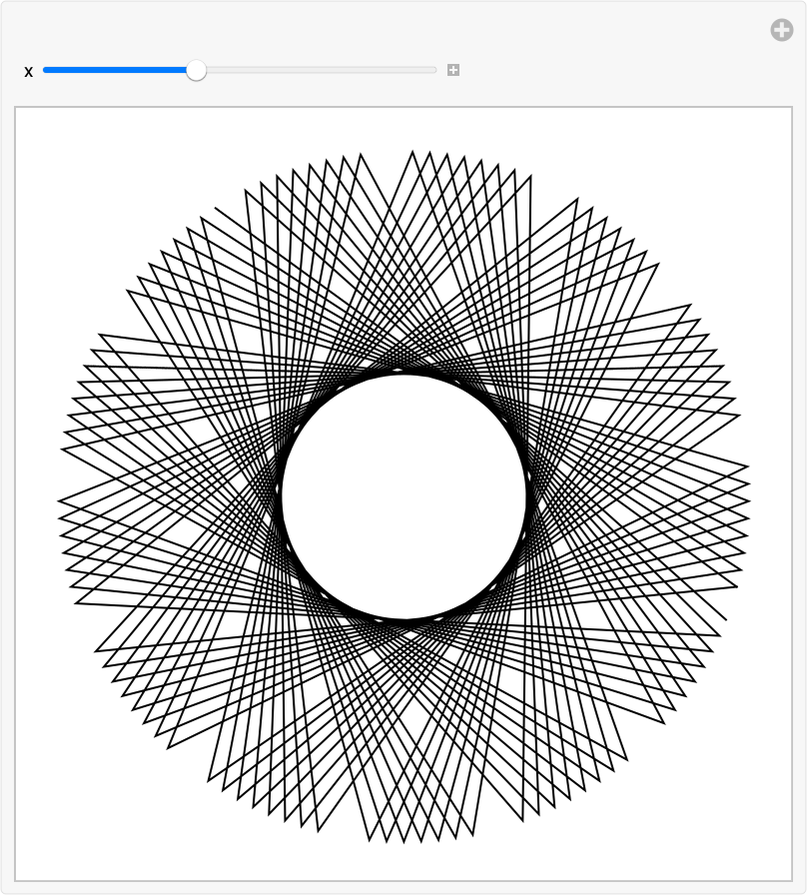Answer & check your solution
17.14Make graphics of the path obtained by successively turning by the digits of 2^10000 multiplied by 30°»
Sample expected output:
 Out[]=Answer & check your solution
+17.1Convert 4.3 light years to furlongs. »
Expected output:
 Out[]=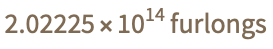Answer & check your solution
+17.2Convert 20,000 leagues to miles. »
Expected output:
 Out[]=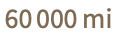Answer & check your solution
+17.3Make a Manipulate to rotate a size-200 “W” to any angle from 0° to 360°»
Sample expected output:
 Out[]=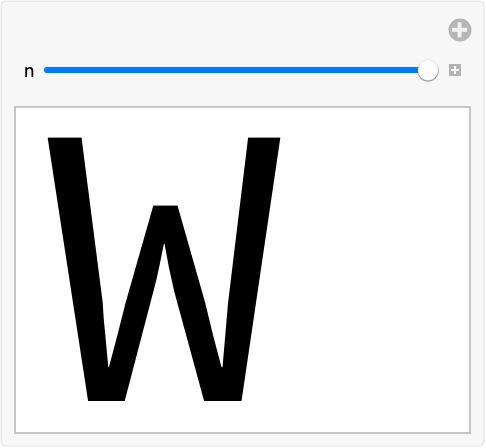Answer & check your solution
+17.4Rotate an image of the Great Pyramid by 180°»
Sample expected output:
 Out[]=Answer & check your solution
+17.5Generate a list of letters of the alphabet, each randomly rotated. »
Sample expected output:
 Out[]=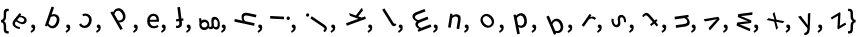Answer & check your solution
+17.6Generate graphics for a path obtained by turning 100 times through a random number of degrees between 0 and 360. »
Sample expected output:
 Out[]=Answer & check your solution
What unit abbreviations does the Wolfram Language understand?
Pretty much any common abbreviation, whether it’s miles/hr or mph or mi/h, etc. (If you’re wondering if an abbreviation will work, just try it.)
Does the Wolfram Language pick units based on what country I’m in?
Yes. For example, it’ll tend to use inches if you’re in the US, and centimeters if you’re in continental Europe.
Do I have to be connected to the network to use units?
Only to interpret input like 5kg. If you type Quantity[5, "Kilograms"] you don’t need the networkexcept to deal with units like currencies whose values are always changing.
What can I do if my unit conversion gives me an exact fraction but I want a decimal number?
Use the function N[...] to find a decimal approximation. Or add a decimal point to a number in your input. We’ll talk more about this in Section 23.
Why don’t I get the same result for the currency conversion example?